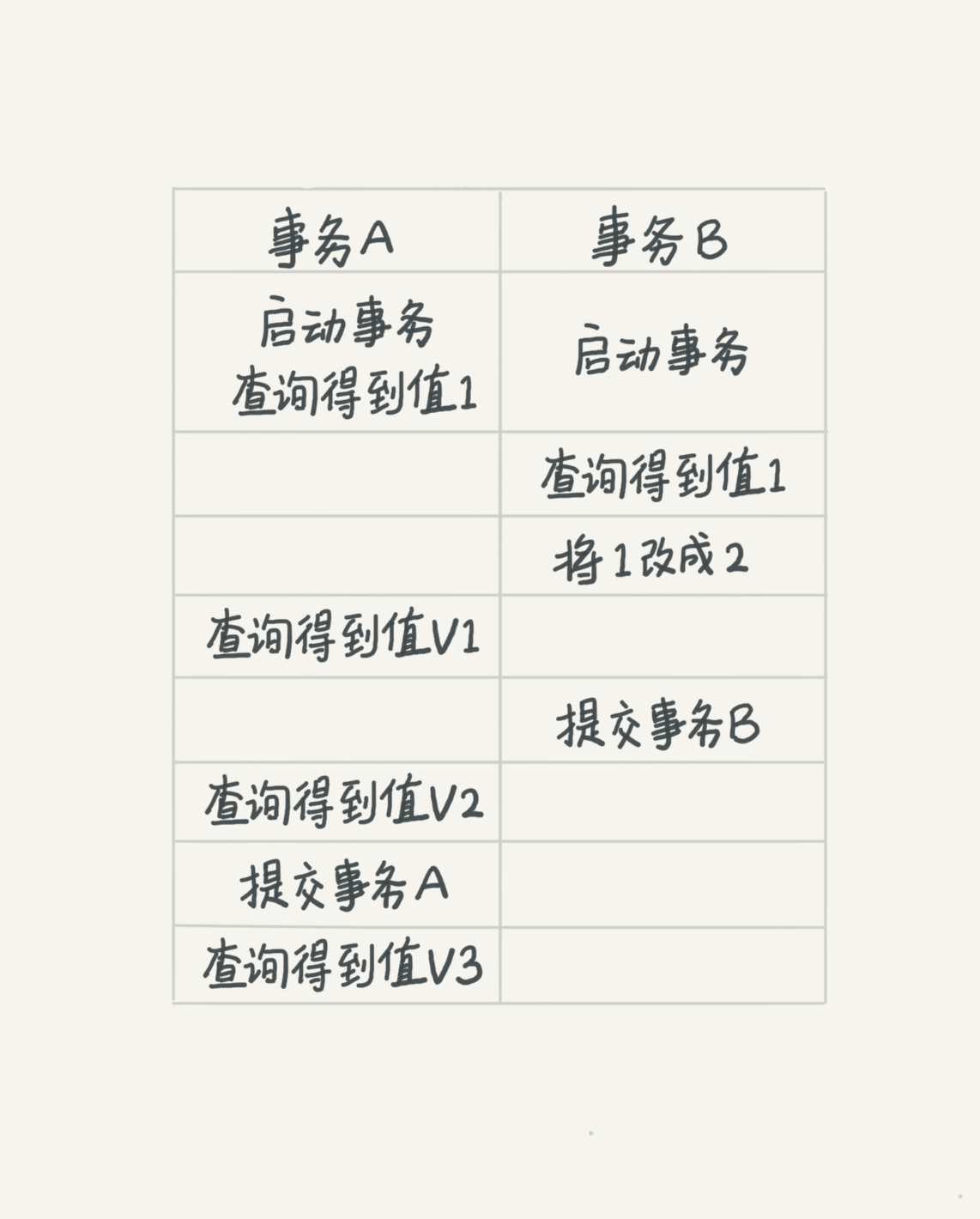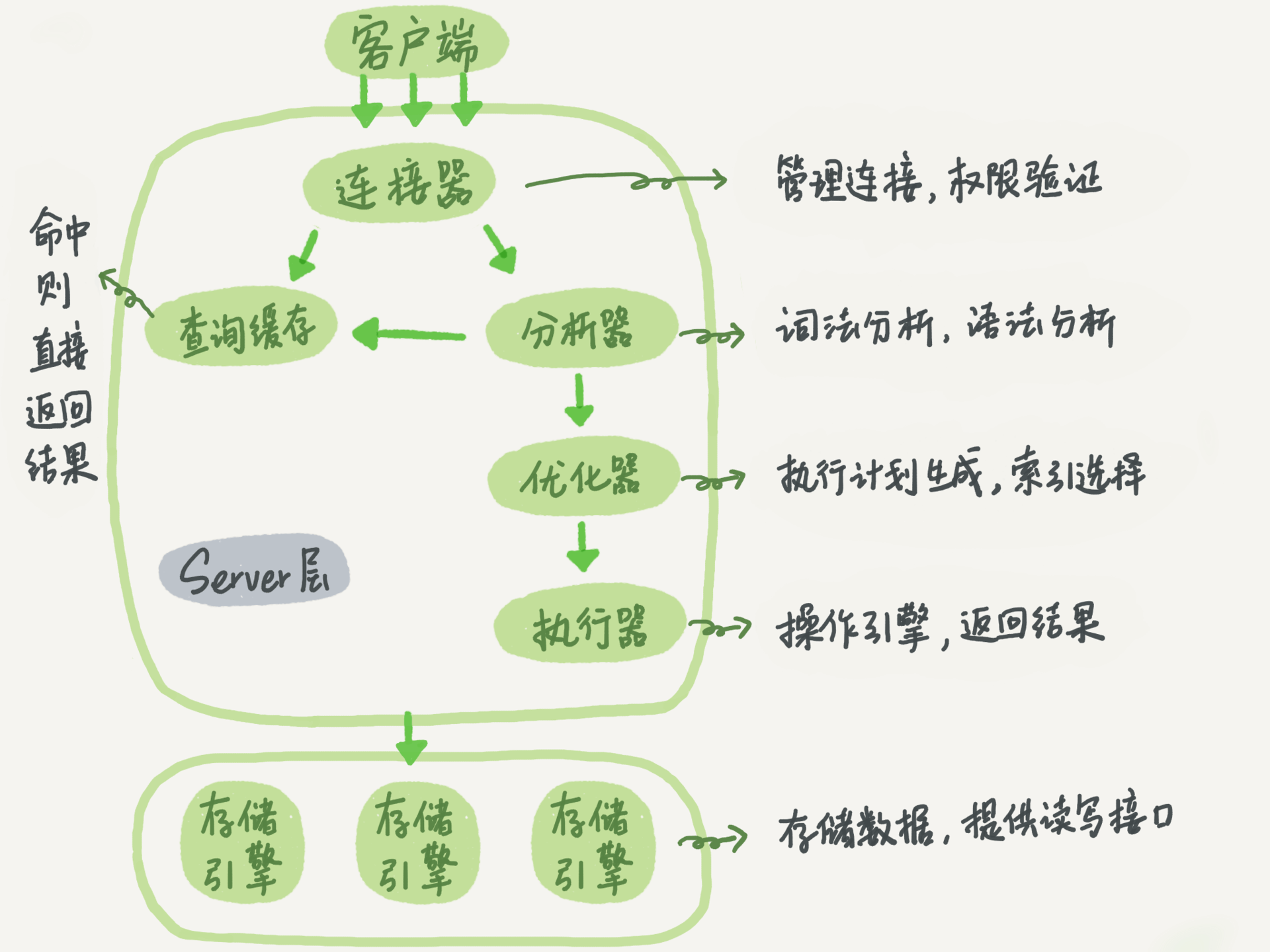5669PayAttentionStarLi9htlzz_9huangbqyxc的小迷妹HfjNUlYZzst_2001MengLeAᴄWingkxzzk8s是对于容器的管理平台，docker 是k8s的组成部分

### k8s官网

https://kubernetes.io/zh-cn/

### 参考链接

https://zhuanlan.zhihu.com/p/53260098 -> 漫画好懂，看几幅k8s结构图就好，大部分都是废话

### wsl

• Windows Subsystem for Linux

• docker windows 版

### 视频教学

https://www.bilibili.com/video/BV11L411g7U1

### 参考

https://blog.csdn.net/lb0737/article/details/80904242

### wsl卸载命令

wsl –unregister Ubuntu-20.04

wsl -l -v

### 事务的性质

• A：Atomicity
• C: Consistency
• I: Isolation
• D: Durability
即原子性、一致性、隔离性、持久性

### 脏读、不可重复读、幻读

• 脏读:读到其他事务未提交的数据
• 不可重复读：前后读取的记录内容不一致
• 幻读：前后读取的记录数量不一致。

### 事务隔离级别

• 串行化（serializable ）

• 读未提交是指，一个事务还没提交时，它做的变更就能被别的事务看到
• 读提交是指，一个事务提交之后，它做的变更才会被其他事务看到
• 可重复读是指，一个事务执行过程中看到的数据，总是跟这个事务在启动时看到的数据是一致的。当然在可重复读隔离级别下，未提交变更对其他事务也是不可见的
• 串行化，顾名思义是对于同一行记录，“写”会加“写锁”，“读”会加“读锁”。当出现读写锁冲突的时候，后访问的事务必须等前一个事务执行完成，才能继续执行。队列，先来后到### 不使用长事务是因为长事务的存在会

1. 占有锁资源，可能拖垮整个库
2. 长事务可能导致暂时需要保存很多undo log，会占用内存空间。

### redo log和binlog

• redo log 是 InnoDB 引擎特有的；binlog 是 MySQL 的 Server 层实现的，所有引擎都可以使用。
• redo log 是物理日志，记录的是“在某个数据页上做了什么修改”；binlog 是逻辑日志，记录的是这个语句的原始逻辑，比如“给 ID=2 这一行的 c 字段加 1 ”。简单的说一个记载的是数据页修改，一个记得是sql语句。
• redo log 是循环写的，空间固定会用完；binlog 是可以追加写入的。“追加写”是指 binlog 文件写到一定大小后会切换到下一个，并不会覆盖以前的日志。• 存储引擎
• InnoDB
• MyISAM
• Memory 等

mysql有多个存储引擎，常见的为InnoDB,默认也是

CREATE TABLE hui_user (
xxx
) ENGINE=InnoDB AUTO_INCREMENT=22 DEFAULT CHARSET=utf8;

hh,没用过后面的参数，笑死

- 参数解释
- ENGINE： 可走默认InnoDB
- AUTO_INCREMENT：开始id
- CHARSET: my.ini 设置过mysql语言编码，这里是设置表单编码



mysql -h$ip -P$port -u\$user -p

MySQL不能确定是用户名不正确还是密码不正确。防破解

mysql 会在没有操作的情况下（8小时）断开连接，由参数 wait_timeout 控制，有没有人试试，头一次见，6

mysql> show variables like ‘wait_timeout’;

Lost connection to MySQL server during query

// 短连接

// 长连接



可以使用定时超时断开，或通过重新初始化链接来清空

mysql5 sql语句查询后经过更新数据操作后缓存失效，mysql8都给它删除了都，没有缓存查询这步

sql语法分析

1. 识别传进来的字符串
2. 根据传进来的字符串用语法规则进行判断
3. 如果有错误，将会收到You have an error in your SQL syntax的报错

1. 分析器进行的权限验证叫做 precheck
2. 执行器进行的权限验证叫做 权限验证，hh,不知道叫啥

1. 连接器会鉴权用户是否具有连接 MySQL 实例的权限，包括用户的账号和密码是否正确，以及用户是否被授予连接权限。
2. 分析器会鉴权用户是否具有对所请求的数据库和表的查询权限，包括是否具有查询、插入、更新、删除等操作的权限，以及是否具有访问某些列的权限。
3. 执行器会鉴权用户是否具有执行所请求的操作的权限，包括对表的读、写等操作权限，以及是否具有执行存储过程和触发器的权限。

#include <iostream>
#include <cstring>
#include <algorithm>

using namespace std;

char get(int x){
if (x <= 9) return x + '0';
return x - 10 + 'A';
}

bool check(string s){
for(int i = 0, j = s.size() - 1;i < j;i ++,j --){
if(s[i] != s[j])
return false;
}
return true;
}

string base(int a,int b){
string num;
while(a) num += get(a % b), a /= b;
reverse(num.begin(), num.end());
return num;
}

int main()
{
int n;
cin >> n;

for(int i = 1; i <= 300; i ++){
auto num = base(i * i, n);
if(check(num))
cout << base(i, n) << " " << num << endl;
}
}


#include <iostream>
#include <cstring>
#include <algorithm>
#include <queue>

using namespace std;

typedef pair<int, int> PII;
const int N = 25;

int n, m;
char g[N][N];

int bfs(int sx,int sy){

queue<PII> q;
q.push({sx, sy});
g[sx][sy] = '#';

int res = 0;

int dx[] = {-1, 0, 1, 0}, dy[] = {0, 1, 0, -1};

while(q.size()){
auto t = q.front();
q.pop();
res ++;

for(int i = 0 ; i < 4; i ++){
int x = t.first + dx[i], y = t.second + dy[i];
if(x < 0 || x >= n || y < 0 || y >= m || g[x][y] != '.')
continue;
g[x][y] = '#';
q.push({x, y});
}
}
return res;
}

int main()
{
while (cin >> m >> n, n || m)
{
for (int i = 0; i < n; i ++ )
cin >> g[i];

int x, y;

for (int i = 0; i < n; i ++ )
for (int j = 0; j < m; j ++ )
if (g[i][j] == '@')
{
x = i;
y = j;
}
cout << bfs(x, y) << endl;
}

return 0;
}


#include <iostream>
#include <algorithm>
#include <cstring>

using namespace std;

const int N = 510;
const int INF=0x3f3f3f3f;

int q[N][N];
int f[N][N];
int n;

int main(){

cin >> n;

for(int i = 1; i <= n; i ++)
for(int j = 1 ; j <= i; j ++)
cin >> q[i][j];

memset(f, -INF, sizeof f);

// f 和 f 是没有的，推导不出来
f = q;
for(int i = 2 ; i <= n; i ++)
for(int j = 1 ; j <= i; j ++)
f[i][j] = max(f[i - 1][j - 1],f[i - 1][j]) + q[i][j];

int res = -INF;

for(int i = 1 ; i <= n; i ++){
res = max(res,f[n][i]);
}

cout << res << endl;
return 0;
}#include <iostream>
#include <cstring>
#include <algorithm>

using namespace std;

int main(){
int n;
cin >> n;

int res1 = 1,res2 = 1e9;

while (n -- ){
int a,b;
cin >> a >> b;

res1 = max(res1, a / (b + 1) + 1);
res2 = min(res2, a / b);
}
cout << res1 << " " << res2 << endl;
return 0;
}